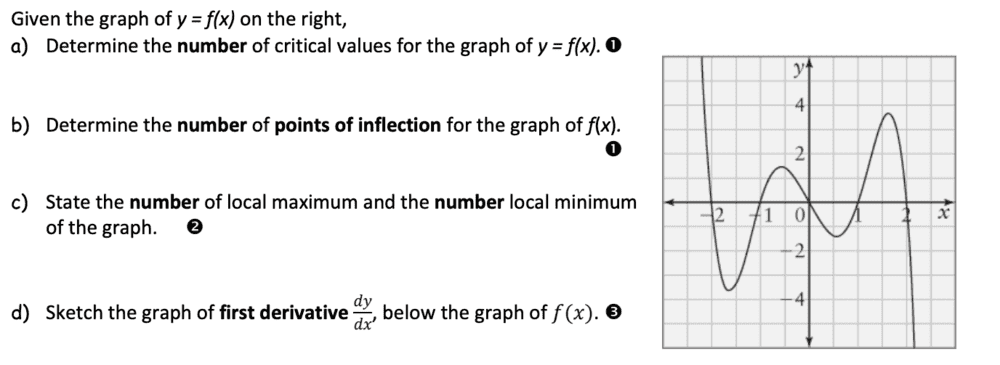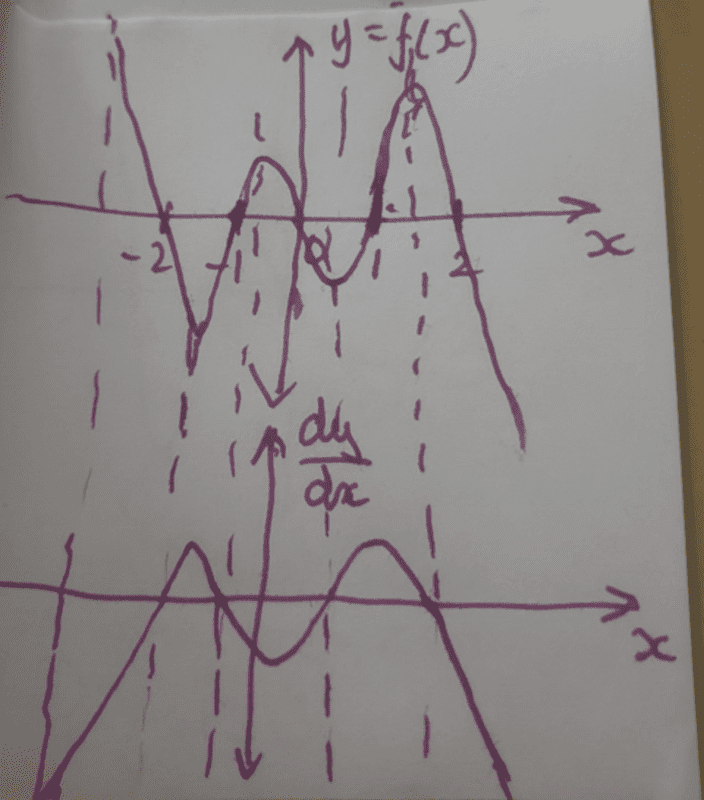# Curve sketching (discussion)

Homework Statement:
The graph (shown below) asks for critical values, the number of points of inflection, local max and min, and the sketch of the first derivative. Can someone let me know if it's right, especially the graph?
Relevant Equations:
n/aa.) 4 critical values
b.) there are no points of inflection
c.) 2 local maxes and mins
d.)LCKurtz
Homework Helper
Gold Member
How do you get your answer for b? What happens at an inflection point?
For c, do you mean 2 local maxes and mins, or 2 of each local maxes and mins? Which is correct?

How do you get your answer for b? What happens at an inflection point?
For c, do you mean 2 local maxes and mins, or 2 of each local maxes and mins? Which is correct?
for b.) the inflection is where a point on a continuous plane curve at which the curve changes from being concave to convex, or vice versa, so I guess instead of 0, there are 2.
as for c.) there are two maximum and two minimum points

haruspex
Homework Helper
Gold Member
2020 Award
instead of 0, there are 2.
Keep looking.

Keep looking.
there's 4.

LCKurtz
Homework Helper
Gold Member
there's 4.
Inflection points? Really?

etotheipi
angela107 said:
there are 2.

there's 4.

... 6, 8, who do we appreciate?

•2milehi
Be careful!
There are 2 types of critical values. First type are the ones that makes ##f'(x)=0## or where the first derivative doesn't exist. Second type are the ones that makes ##f''(x)=0## or where the second derivative doesn't exist.
First type critical values could be extremes (maximum or minimum) or could not, and second type could be points of inflections or could not.
Here you don't have the expression of ##f(x)## so we'll work with what we see in the graph.
Local maximum ##x_0## are the ones that makes ##f(x)<=f(c)## in a neighbourhood of ##x_0##.
Local minimum ##x_0## are the ones that makes ##f(x)>=f(c)## in a neighbourhood of ##x_0##.
Points of inflections are the ones in which the concavity changes its direction.
And we could treat critical values as the sum of local extremes and points of inflection because the curve is smooth. You can see that there are no angled points, where the first derivative doesn't exist. Those point would be added to the critical values if existed.